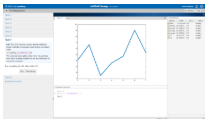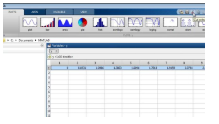Main Content

# Get Started with MATLAB

The Language of Technical Computing

Millions of engineers and scientists worldwide use MATLAB® to analyze and design the systems and products transforming our world. The matrix-based MATLAB language is the world’s most natural way to express computational mathematics. Built-in graphics make it easy to visualize and gain insights from data. The desktop environment invites experimentation, exploration, and discovery. These MATLAB tools and capabilities are all rigorously tested and designed to work together.

MATLAB helps you take your ideas beyond the desktop. You can run your analyses on larger data sets, and scale up to clusters and clouds. MATLAB code can be integrated with other languages, enabling you to deploy algorithms and applications within web, enterprise, and production systems.

## Tutorials

• Desktop Basics

Enter statements at the command line and view results.

• Matrices and Arrays

MATLAB operates primarily on arrays and matrices, both in whole and in part. A matrix is a two-dimensional array often used for linear algebra.

• Array Indexing

Variables in MATLAB are typically arrays that can hold many numbers. When you want to access selected elements of an array, use indexing.

• Workspace Variables

The workspace contains variables that you create within or import into MATLAB from data files or other programs.

• Text and Characters

Create string arrays for text, or create character arrays for data.

• Calling Functions

MATLAB provides a large number of functions that perform computational tasks. To call a function, enclose its input arguments in parentheses.

• 2-D and 3-D Plots

Graphics functions include 2-D and 3-D plotting functions to visualize data and communicate results.

• Programming and Scripts

The simplest type of MATLAB program is called a script. A script contains a sequence of commands and function calls.

• Help and Documentation

All functions have supporting documentation that includes examples and describes the function inputs, outputs, and calling syntax.

## Interactive LearningMATLAB Onramp
Free two-hour online MATLAB course

## VideosGetting Started with MATLAB
Get an overview of MATLAB, the language of technical computing.Working in the Development Environment
Access tools such as the command history workspace browser and variable editor, save and load your workspace data, and manage windows and desktop layout.

Download ebook